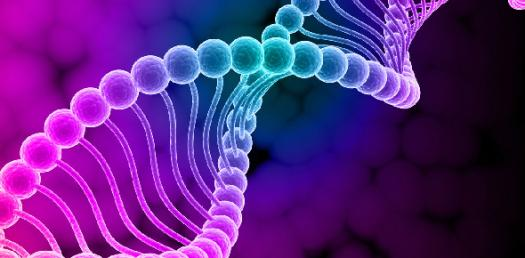# Genetics Test For Students! Trivia Quiz

35 Questions | Total Attempts: 235SettingsAs humans, we are a lot similar than we may think as in the world today, there is someone who has the same genetic material as you do. Did you know that humans and mice genetics are not that different? This test is the final one before you take your main exams. Take it up and get to see which chapters you need to spend a little more time on. All the best!

• 1.
If a frog's cell has 26 chromosomes, then its sex cells must have________chromosomes.
• A.

13

• B.

52

• C.

26

• D.

32

• 2.
Gametes or sperm and egg are produced by the process of:
• A.

Meiosis

• B.

Mitosis

• C.

Fertilization

• D.

Complete dominance

• 3.
A trait that is the “weaker” of two traits is called
• A.

Incomplete

• B.

Dominant

• C.

Recessive

• D.

Heterozygous

• 4.
A stronger trait or allele is
• A.

Incomplete

• B.

Dominant

• C.

Recessive

• D.

Heterozygous

• 5.
The visible characteristics of an organism are called.
• A.

Cells

• B.

Genotype

• C.

Chromosome

• D.

Phenotype

• 6.
If two dragons that are “Tt” for tail genes are crossed, what percentage of their offspring will most likely be “Tt” (Hint: Use a Punnett Square)
• A.

25

• B.

50

• C.

75

• D.

100

• 7.
When one allele in a pair totally dominates another allele, the gene is said to show:
• A.

Heterozygous

• B.

Complete dominance

• C.

Incomplete dominance

• D.

Meiosis

• 8.
DNA is:
• A.

The code that allows for different forms of genes

• B.

The chemical code that is made from A,T,G,C

• C.

A genetic code that genes and chromosomes are made from.

• D.

All of the above

• 9.
What structure is "A"?
• A.

Cell

• B.

Nucleus

• C.

Chromosome

• D.

Mitochondria

• E.

Gamete

• 10.
When the genes in a gene pair appear to be blending together, the genes are said to show:
• A.

Heterozygous

• B.

Complete dominance

• C.

Incomplete dominance

• D.

Meiosis

• 11.
What is structure "B"?
• A.

Cell

• B.

Nucleus

• C.

Chromosome

• D.

Mitochondria

• E.

Gamete

• 12.
What is structure "C"?
• A.

Cell

• B.

Nucleus

• C.

Chromosome

• D.

Mitochondria

• E.

Gamete

• 13.
Which of the following is true?
• A.

Chromosomes are located on genes of an organism

• B.

There are a total of 23 chromosomes in the human body.

• C.

Chromosomes contain genes.

• D.

Chromosomes are another more scientific way to say genes.

• 14.
The basic units that pass on traits are called:
• A.

Genes

• B.

Cells

• C.

Mitochondria

• D.

Phenos

• E.

Nucleus

• 15.
Give an example of a heterozygous genotype.
• A.

RR

• B.

Rr

• C.

Rr

• D.

R

• E.

R

• 16.
When a portion of the DNA is removed, it is this type of mutation.
• A.

Secretion

• B.

Deletion

• C.

Insertion

• D.

Inversion

• E.

Verizon

• 17.
Give an example of a homozygous recessive genotype.
• A.

HH

• B.

Hh

• C.

Hh

• D.

H

• E.

H

• 18.
This structure is a called:
• A.

DNA

• B.

Meiotic Fractionation

• C.

Smooth ER

• D.

Rough ER

• E.

Amino Replicates

• 19.
The nitrogen bases that make up this structure are:
• A.

AGCV

• B.

AGVT

• C.

VCTA

• D.

AGCT

• 20.
What is structure "A"?
• A.

Cell

• B.

Chromosome

• C.

Gene

• D.

Meiotic Cells

• 21.
What is structure "B"?
• A.

Cell

• B.

Chromosome

• C.

Gene

• D.

Meiotic Cells

• 22.
“Hh” is an example of an organism’s
• A.

Genotype

• B.

Chromosome

• C.

Phenotype

• D.

Pedigree

• E.

Punnet square

• 23.
The dragon has purple ears is an example of a dragon's:
• A.

Genotype

• B.

Chromosome

• C.

Phenotype

• D.

Pedigree

• E.

Punnet square

• 24.
If Two dragons that are “Tt” for tail genes are crossed and "T" is completely dominant over "t", what percentage of their offspring will most likely have no spikes.   ( TT - spikes   Tt - spikes   tt- no spikes)
• A.

0

• B.

25

• C.

50

• D.

75

• E.

100

• 25.
If red flower color “R” is incompletely dominant over white flower color “r”, then what would be the color of an individual that has genes that are “R r”
• A.

Red

• B.

White

• C.

Pink

• D.

Black

Related TopicsBack to top